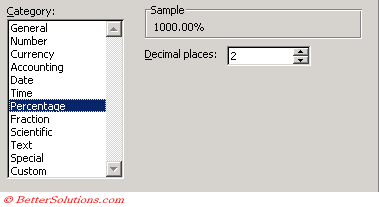# Percentage

This format multiplies the value in the cell by 100 and displays the result with a percent sign after it.Decimal places - The number of decimal places is from 0 to 30.

This displays fractional numbers as percentages.
For example 0.5 is translated into 50%
You can choose the number of decimal places
If you forget to start your number with a decimal point, Excel will correct this for you automatically.
If you enter the number 3 and then format the cell as a percentage it is displayed as 300%.
If you format the cell as a percentage first and then enter the number 3, this is displayed as 3%

This means that you can't enter any decimals to represent percentages larger than 100%
If the cell is formatted as a percentage to enter 200% you must type 200 and not 2.

This displays numbers as percentages.
Any decimal place is moved two places to the right and a percent sign is appended to the end of the number.

### Percent Style

This number format can be quickly applied by pressing the following button.
Percent Style - Displays the number as a percentage by applying the format "0%".